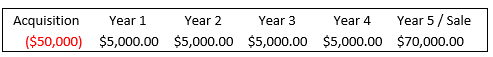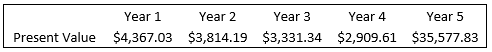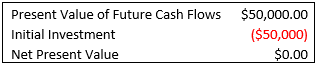# Breaking Down IRR in Real EstateBy Ryan Voccia (J&G Student Since March 2020)

If you invest \$50,000 into a real estate deal that goes on to produce \$90,000 of income during the period you held property, would you consider that a good investment?

When you purchase investment real estate, you are paying for a stream of income. You likely expect the property to generate cash flow and later be sold at a higher price than you paid. That income comes in monthly intervals as residents pay rent and then again at the sale of the property. Your cash flows may add up to look like something in the example below.A common return metric used to evaluate these cash flows is the Internal Rate of Return, or IRR.

The IRR is the discount rate that makes the net present value of all future cash flows equal zero in a discounted cash flow model. That may sound like a lot of jargon, so let’s break this down to understand better what IRR means.

One of the most important things to understand is that IRR is a time value of money calculation. A dollar earned from a cash flow stream in year one has more value than that same dollar earned in year two. Why? The sooner we earn income, the sooner that income can be reinvested. Using the cash flow example listed above, the present value (value in today’s dollars) of the cash flow in year one is greater than the present value of cash flow received in year two.To determine the present value of a projected future cash flow, you need to discount the future cash flows back to the present. You do this by using a discount rate. For the purposes of discounted cash flow modeling, the discount rate you choose should be your required rate of return for a similar asset with a similar risk profile.

After calculating the present value of all future cash flows in our example, we can add them all up to determine the present value of the total investment. We can then subtract our initial investment from the present value of future cash flows to get our Net Present Value (NPV).If your NPV is greater than zero, your rate of return is greater than your discount rate. Remember, the IRR is the discount rate that makes NPV equal to zero. Below is the IRR of the example we have been using.Let’s go back and do a discounted cash flow analysis using 14.49% as our discount rate.Below you can see that the NPV is equal to zero when using a discount rate of 14.49%.Why does the timing of cash flows matter? Let’s look at the two examples below. The first one is the same example we have been using. The second example does not assume any cash flow until the property is sold at year 5. You can see that the total return for both properties is the same; however, the second example has a lower IRR. Why? In example one, rental income received in years 1-4 could be reinvested. In example two, your money is tied up in the project until the sale. There was no opportunity to reinvest capital during those five years.Conclusion:

Going back to the question posed at the beginning of the article, was your initial \$50,000 dollar investment a good investment? It depends. One of the first questions you should ask is at what intervals you will be receiving your return and over what time frame. Internal rate of return is a return metric that we can use to help us evaluate a potential investment. Used in conjunction with cash-on-cash, equity multiple, and average annual return, IRR can help analyze a deal’s return metrics. If there are any questions or comments, please comment below or feel free to contact me at ryan@seasideinvests.com . Thank you for reading.